# NCERT Solutions for Class 6 Maths Chapter 5 Understanding Elementary Shapes (Ex 5.9) Exercise 5.9

## NCERT Solutions for Class 6 Maths Chapter 5 Understanding Elementary Shapes (Ex 5.9) Exercise 5.9

Free PDF download of NCERT Solutions for Class 6 Maths Chapter 5 Exercise 5.9 (Ex 5.9) and all chapter exercises at one place prepared by expert teacher as per NCERT (CBSE) books guidelines. Class 6 Maths Chapter 5 Understanding Elementary Shapes Exercise 5.9 Questions with Solutions to help you to revise complete Syllabus and Score More marks. Register and get all exercise solutions in your emails.

Do you need help with your Homework? Are you preparing for Exams?
Study without Internet (Offline)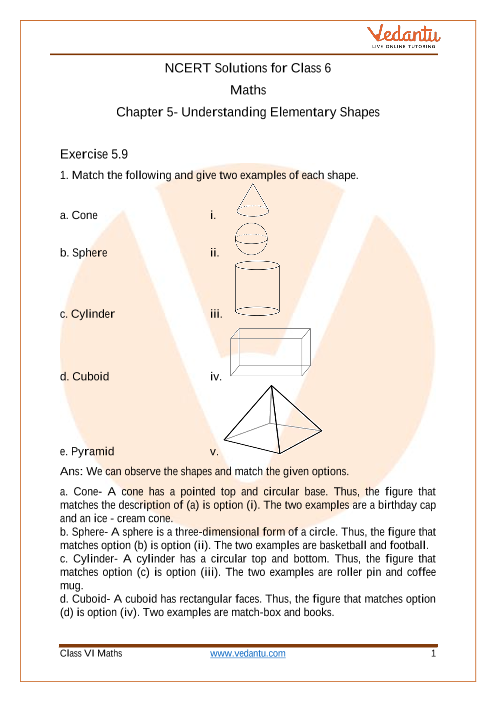Book your Free Demo session
Get a flavour of LIVE classes here at Vedantu## Access NCERT Solutions for Class 6 Mathematics Chapter 5 - Understanding Elementary Shapes

Exercise 5.9

1. Match the following and give two examples of each shape.

a. Cone

i.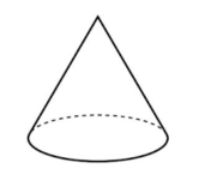b. Sphere

ii.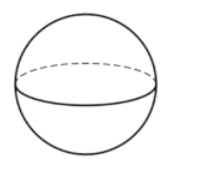c. Cylinder

iii.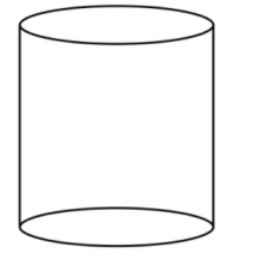d. Cuboid

iv.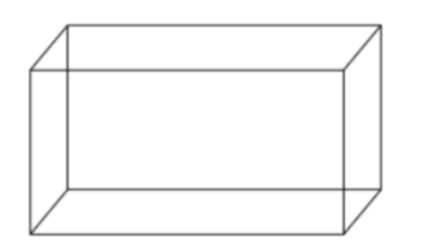e. Pyramid

v.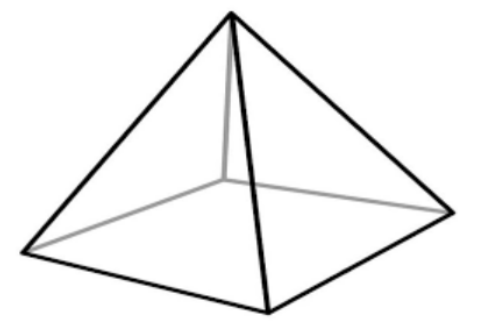Ans: We can observe the shapes and match the given options.

a. Cone- A cone has a pointed top and circular base. Thus, the figure that matches the description of (a) is option (i). The two examples are, a birthday cap and an ice-cream cone.

b. Sphere- A sphere is a three-dimensional form of circle. Thus, the figure that matches option (b) is option (ii). The two examples are basketball and football.

c. Cylinder- A cylinder has a circular top and bottom. Thus, the figure that  matches option (c) is option (iii). The two examples are, roller pin and coffee mug.

d. Cuboid- A cuboid has rectangular faces. Thus, the figure that matches option (d) is option (iv). Two examples are, match-box and books.

e. Pyramid- A pyramid has triangular faces. Thus, the figure that matches option (e) is option (v). Two examples of pyramids are camping tents and Toblerone chocolate.

2. What is the shape of the following items?

Ans: My instrument box is a cuboid.

b. A brick

Ans: A brick is a cuboid.

c. A match box

Ans: A match box is a cuboid.

Ans: The shape of a road roller is a cylinder.

Ans: The shape of a laddu is a sphere.

## NCERT Solutions for Class 6 Maths Chapter 5 Understanding Elementary Shapes Exercise 5.9

Opting for the NCERT solutions for Ex 5.9 Class 6 Maths is considered as the best option for the CBSE students when it comes to exam preparation. This chapter consists of many exercises. Out of which we have provided the Exercise 5.9 Class 6 Maths NCERT solutions on this page in PDF format. You can download this solution as per your convenience or you can study it directly from our website/ app online.

Vedantu in-house subject matter experts have solved the problems/ questions from the exercise with the utmost care and by following all the guidelines by CBSE. Class 6 students who are thorough with all the concepts from the Subject Maths textbook and quite well-versed with all the problems from the exercises given in it, then any student can easily score the highest possible marks in the final exam. With the help of this Class 6 Maths Chapter 5 Exercise 5.9 solutions, students can easily understand the pattern of questions that can be asked in the exam from this chapter and also learn the marks weightage of the chapter. So that they can prepare themselves accordingly for the final exam.

Besides these NCERT solutions for Class 6 Maths Chapter 5 Exercise 5.9, there are plenty of exercises in this chapter which contain innumerable questions as well. All these questions are solved/answered by our in-house subject experts as mentioned earlier. Hence all of these are bound to be of superior quality and anyone can refer to these during the time of exam preparation. In order to score the best possible marks in the class, it is really important to understand all the concepts of the textbooks and solve the problems from the exercises given next to it.

Do not delay any more. Download the NCERT solutions for Class 6 Maths Chapter 5 Exercise 5.9 from Vedantu website now for better exam preparation. If you have the Vedantu app in your phone, you can download the same through the app as well. The best part of these solutions is these can be accessed both online and offline as well.SHARETWEETSHARESUBSCRIBE